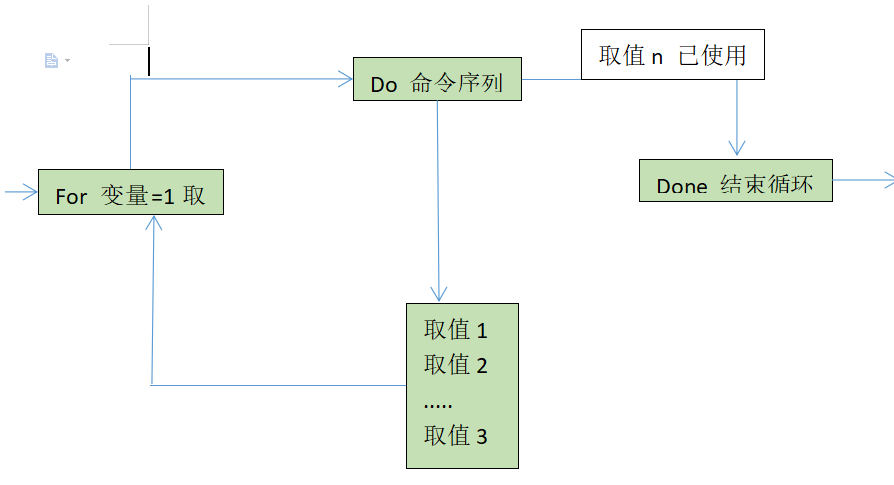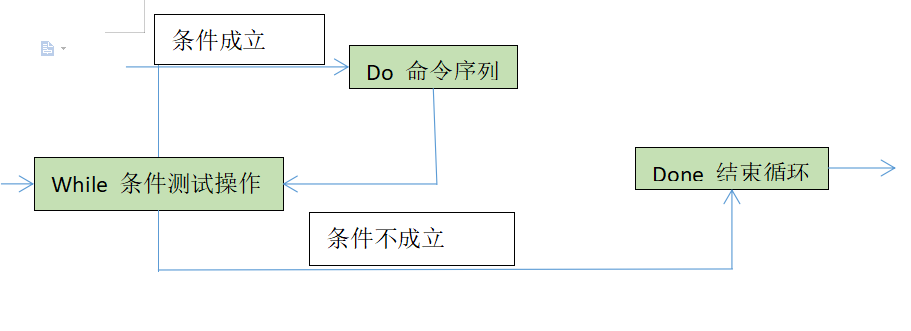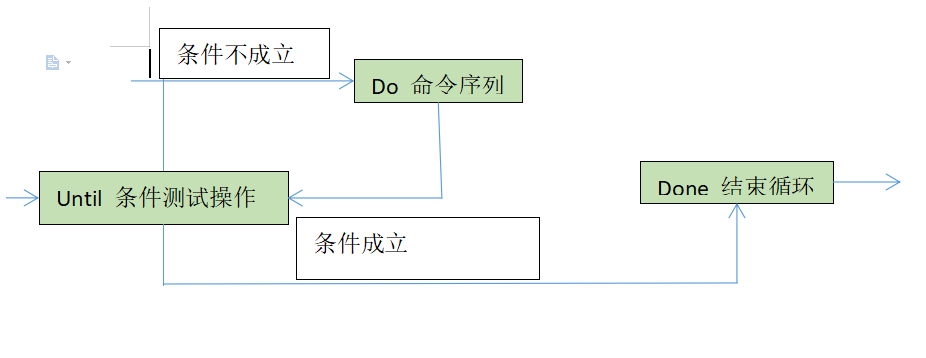# 一、for循环

for循环，又称条件循环，或者for in， 就是for循环的特性，次数和给予的条件是成正比

### for语句的结构

##### 读取不同的变量值，用来逐个执行同一组命令
for  变量名 in  取值列表
do
命令序列
done### 例题

##### 1、输入1-100之间的整数和，
[root@localhost ~]# vim zheng.sh  //输入一个.sh文件
#!/bin/bash  //脚本声明
sum=0     //定义一个变量和
for ((i=1;i<=$a;i++)) //for循环，i=1，循环次数小于等于变量a的值，i每次循环加1 do sum=$[$sum +$i]
done
echo $sum //输出和的值 [root@localhost ~]# bash zheng.sh //通过bash运行zheng.sh文件 请输入一个0-100之间的整数：12 // 当输入12时表示从1-12之间的数相加得出来的数 78 [root@localhost ~]# bash zheng.sh 请输入一个0-100之间的整数：32 528  ###### 2、计算1-100之间的偶数和 [root@localhost ~]# vim s.sh //编辑一个文件 #!/bin/bash //脚本声明 sum=0 for i in {1..100} //数的取值范围 do if [$((i%2)) -eq 0 ];then  //偶数的表达式
sum=$[$i+$sum] fi done echo$sum    //输出和的值
[root@localhost ~]# bash s.sh
2550  //通过bash运行可以查看1-100之间的偶数和值


##### 3、批量添加新的用户

[root@localhost ~]# vim user.txt  //将新建的用户放进user.txt文件中
liu1
liu2
liu3
[root@localhost ~]# vim user,sh  //创建新的.sh
#!/bin/bash
a=cat user.txt  //将user.txt文件直接写入
for i in $a do useradd$i
echo "123" | passwd --stdin $i //修改密码 done [root@localhost ~]# bash user.sh //执行命令 更改用户liu1的密码。 passwd：所有的身份验证令牌已经成功更新。 更改用户liu2的密码。 passwd：所有的身份验证令牌已经成功更新。 更改用户liu3的密码。 passwd：所有的身份验证令牌已经成功更新。  ##### 4、ping网段网站 [root@localhost ~]# vim ping.sh #!/bin/bash ip=192.168.159 for i in {1..254} //设置取值范围 do ping -c -2 -i 2$ip.$i > /dev/null if [$? -eq 0 ];then
echo "$ip.$i 在线"
else
echo "$ip.$i 不在线"
fi
done



5、九九乘法表

[root@localhost ~]# vim haowan.sh
#!/bin/bash
for i in seq 9
do
for j in seq $i do echo -n "$i*$j=$[i*j] "
done
echo
done
[root@localhost ~]# bash  haowan.sh
1*1=1
2*1=2 2*2=4
3*1=3 3*2=6 3*3=9
4*1=4 4*2=8 4*3=12 4*4=16
5*1=5 5*2=10 5*3=15 5*4=20 5*5=25
6*1=6 6*2=12 6*3=18 6*4=24 6*5=30 6*6=36
7*1=7 7*2=14 7*3=21 7*4=28 7*5=35 7*6=42 7*7=49
8*1=8 8*2=16 8*3=24 8*4=32 8*5=40 8*6=48 8*7=56 8*8=64
9*1=9 9*2=18 9*3=27 9*4=36 9*5=45 9*6=54 9*7=63 9*8=72 9*9=81



# 二、while 循环

### while语句结构

while    条件测试操作
do
命令序列
done##### 1、输出1到10
[root@localhost ~]# vim chengfa.sh
#!/bin/bash
num=1
while [ $num -le 10 ] do echo$num
let num++
done
[root@localhost ~]# bash chengfa.sh
1
2
3
4
5
6
7
8
9
10


##### 2、求1-100之间的和是多少
[root@localhost ~]# vim zs.sh
#!/bin/bash
i=1
sum=0
while [ $i -le 100 ] do let sum=$i+$sum let i++ done echo$sum
[root@localhost ~]# bash  zs.sh
5050

##### 3、求1-100之间的偶数的和
[root@localhost ~]# vim os.sh
#!/bin/bash
i=1
sum=0
while [ $i -le 100 ] do if [$((i%2)) -eq 0 ];then
let sum=$i+$sum
fi
let i++
done
echo  $sum [root@localhost ~]# bash os.sh 2550  ##### 4、猜数字游戏，当没有猜对一直循环猜数 [root@localhost ~]# vim chengfa.sh #!/bin/bash n=30 while true do read -p "请输入一个数字：" num if [$num -eq $n ];then echo "你猜对了" break elif [$num -gt $n ];then echo "你猜大了，在小点" elif [$num -lt $n ];then echo "你猜小了，在往大点的猜" fi done [root@localhost ~]# bash chengfa.sh 请输入一个数字：10 你猜小了，在往大点的猜 请输入一个数字：40 你猜大了，在小点 请输入一个数字：30 你猜对了  # 三、until循环 重复测试某个条件，只要条件不成立则反复循环 ### until语句结构 until 条件测试操作 do 命令序列 done##### 1、计算1~50的和 [root@localhost ~]# vim until_1~50.sh #!/bin/bash sum=0 i=1 until [$i -gt 50 ]
do
sum=$[$sum + $i ] let i++ done echo "1~50的和为$sum"
[root@localhost ~]# bash  until_1~50.sh
1~50的和为1275



# 四、常用转义字符

echo -n  表示不换行输出
echo -e  输出转义字符，将转义后的内容输出到屏幕上

\c 不换行输出，在“\c”后面不存在字符的情况下，作用相当于 echo -n；但是当“\c”后面仍然存在字符时，“\c”后面的字符将不会被输出。
\n 换行，被输出的字符从“\n”处开始另起一行。
\f 换行，但是换行后的新行的开头位置连接着上一行的行尾；
\v 与\f相同；
\t 转以后表示插入tab，即横向制表符；
\r  光标移至行首，但不换行，相当于使用“\r”以后的字符覆盖“\r”之前同等长度的字符；但是当“\r”后面不存在任何字符时，“\r”前面的字符不会被覆盖
\\ 表示插入“\”本身；

10-1827万+
06-0647
10-03370
04-28750
03-1416万+
05-13155
04-094138
12-203585
05-013351
09-103354
11-04371
12-29252
12-06202
12-23565
04-21303
03-10755
10-22307
07-071708

### “相关推荐”对你有帮助么？

•非常没帮助
•没帮助
•一般
•有帮助
•非常有帮助被折叠的  条评论 为什么被折叠?到【灌水乐园】发言L先生@..

¥2 ¥4 ¥6 ¥10 ¥20余额支付 (余额：-- )扫码支付获取中扫码支付点击重新获取扫码支付1.余额是钱包充值的虚拟货币，按照1:1的比例进行支付金额的抵扣。
2.余额无法直接购买下载，可以购买VIP、C币套餐、付费专栏及课程。余额充值